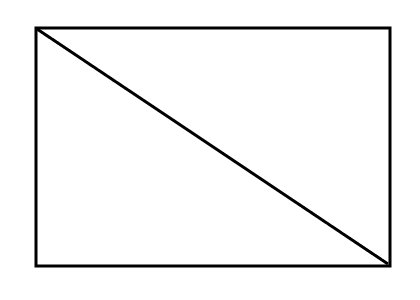SEARCH HOMEMath Central Quandaries & QueriesQuestion from Alfred, a student: How do I find the sum of the interior angles of an 18-sided polygon?Hi Alfred,
It is a common fact that the sum of the interior angles of a triangle is 180 degrees. If you find out how many triangles you can make using the vertices of the polygon, you can easily figure out the sum of the interior angles. Consider a rectangle:You can use two triangles to make a rectangle which is two less than the number of sides. A pentagon can be made by 3 triangles, a hexagon can be made by 4 triangles, etc. To find the sum of the interior angles for any polygon use (n-2)*180 degrees where n is the number of sides.

JaniceMath Central is supported by the University of Regina and The Pacific Institute for the Mathematical Sciences.